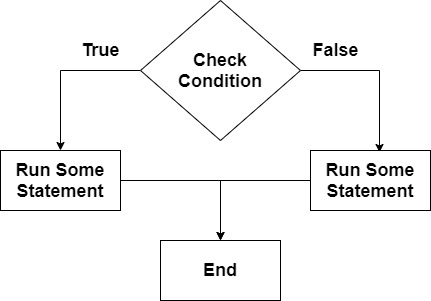# Python if, if and else

Decision-making is a very important part of programming. Every programing language has a decision-making statement that helps the programmer make decision-making tasks. In Python if, if …​ else, and nested if …​ else statements are used for decision-making tasks. Let’s explore those statements.

## Objectives

• Try to understand how the if else statements are work

• If statements

• If else statements

• Nested If else statements

## Try to understand how the Python if else statements areThe above flowchart showing that, first it’s checking a condition, if the condition is true then it will execute some sort of codes or statement and if it false then will run different codes or statement, finally end the whole process.

If you try to understand the flow by a practical example. If today is Friday then it will holiday, if not friday then need to go to school or work.

## Python If statements

Syntax

``````if test condition:
true statement(s)``````

Code

``````# If condition
age = 25
if age >= 18:

Output

``````Hmm, You are adult.

Process finished with exit code 0``````

## Python If else statements

Syntax

``````if test condition:
true statement(s)
else:
false statement(s)``````

Code

``````# If else condition
age = 16
if age >= 18:
else:

Output

``````You are not adult.

Process finished with exit code 0``````

## Python Nested If else statements

Syntax

``````if test condition:
statement(s)
elif test condition:
statement(s)
else:
statement(s)``````

Code

``````# Nested If else condition
age = 18
if age < 2:
print("You are baby.")
elif age < 11:
print("You are children.")
elif age < 18:
``````You are adult.